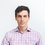# Ohmic sap flow through trees

We're now in a position to model the entire flow of sucrose, from leaf to root. As we've established, the hydraulic flow across the xylem:phloem boundary, as well as down the phloem in the trunk, can be modeled as a given pressure giving rise to some flow, related by an effective resistivity $\mathcal{R}$ just as in electronic circuits.

In fact, we can take the analogy further.

The water loads the phloem from the xylem in the leaf. From here, it moves into the phloem of the trunk, and flows on to the sites of sucrose consumption, all the while riding the same osmotic pressure gradient.

This pressure across the vascular system is equivalent to a potential voltage kept across a circuit. Indeed, we can say that the xylem:phloem membrane, and the phloem are in series with one another, each opposing the flow of sap.

We can therefore calculate the fluid flow as if we have two resistors (one for the membrane and one for the stem) in series with a battery (the osmotic pressure):

$\Delta p = v_{sap}(\mathcal{R}_{mem} + \mathcal{R}_{stem})$

This idea is illustrated in the figure below:As $\mathcal{R}_{mem}(l)$ is a function of leaf length and $\mathcal{R}_{stem}(h)$ is a function of tree height, we have an expression for the sap flow speed in terms of the size of the leaf $l$ and the height of the tree $h$:

\begin{aligned} \displaystyle v_{sap} &= \frac{\Delta p}{(\mathcal{R}_{mem} + \mathcal{R}_{stem})} \\ &= \frac{\Delta p}{\frac{8h\eta}{\pi R^4} + \frac{1}{2\pi R l L_p}} \\ &= \frac{2 L_p R^4\pi l \Delta p}{R^3 + 16 h \eta l} \end{aligned}

From here, we can take limiting cases and obtain simple expressions for interesting quantities such as the range possible of leaf sizes for a tree of a given height. Perhaps more interestingly, we can solve for the maximum possible height that a tree can have.

As the form indicates, the maximum possible sap flow rate is a saturating (i.e. asymptotic) function of leaf length, $l$. In theory, we can reach this point by having infinitely large leaves. However, building leaves itself costs energy, and so, at some point, we experience diminishing returns with increased leaf size.leaf sizes

In the other direction, smaller leaves result in lower sucrose flow speeds and, at some point, the flow so generated fails to provide any advantage over theoretical plants of the same height without any vascular system, i.e. plants that depend solely on diffusion for sugar transport. It stands to reason that any circulatory system would have to at least outperform such theoretical plants to justify its usefulness.

Using this insight, we can calculate the range of possible leaf sizes as a function of a tree's height as well as the molecular details of the phloem and sap.

Next, let's calculate the maximum possible leaf size as a function of tree height.

Illustration by Maxicat RhododendronNote by Josh Silverman
7 years, 1 month ago

This discussion board is a place to discuss our Daily Challenges and the math and science related to those challenges. Explanations are more than just a solution — they should explain the steps and thinking strategies that you used to obtain the solution. Comments should further the discussion of math and science.

When posting on Brilliant:

• Use the emojis to react to an explanation, whether you're congratulating a job well done , or just really confused .
• Ask specific questions about the challenge or the steps in somebody's explanation. Well-posed questions can add a lot to the discussion, but posting "I don't understand!" doesn't help anyone.
• Try to contribute something new to the discussion, whether it is an extension, generalization or other idea related to the challenge.
• Stay on topic — we're all here to learn more about math and science, not to hear about your favorite get-rich-quick scheme or current world events.

MarkdownAppears as
*italics* or _italics_ italics
**bold** or __bold__ bold
- bulleted- list
• bulleted
• list
1. numbered2. list
1. numbered
2. list
Note: you must add a full line of space before and after lists for them to show up correctly
paragraph 1paragraph 2

paragraph 1

paragraph 2

[example link](https://brilliant.org)example link
> This is a quote
This is a quote
    # I indented these lines
# 4 spaces, and now they show
# up as a code block.

print "hello world"
# I indented these lines
# 4 spaces, and now they show
# up as a code block.

print "hello world"
MathAppears as
Remember to wrap math in $$ ... $$ or $ ... $ to ensure proper formatting.
2 \times 3 $2 \times 3$
2^{34} $2^{34}$
a_{i-1} $a_{i-1}$
\frac{2}{3} $\frac{2}{3}$
\sqrt{2} $\sqrt{2}$
\sum_{i=1}^3 $\sum_{i=1}^3$
\sin \theta $\sin \theta$
\boxed{123} $\boxed{123}$

## Comments

There are no comments in this discussion.

×

Problem Loading...

Note Loading...

Set Loading...